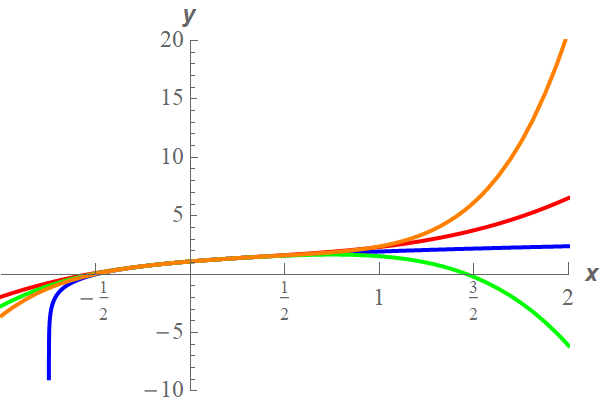Paul's Online Notes
Home / Calculus II / Series & Sequences / Applications of Series
Show Mobile Notice Show All Notes Hide All Notes
Mobile Notice
You appear to be on a device with a "narrow" screen width (i.e. you are probably on a mobile phone). Due to the nature of the mathematics on this site it is best views in landscape mode. If your device is not in landscape mode many of the equations will run off the side of your device (should be able to scroll to see them) and some of the menu items will be cut off due to the narrow screen width.

### Section 10.17 : Applications of Series

3. Write down $${T_3}\left( x \right)$$, $${T_4}\left( x \right)$$ and $${T_5}\left( x \right)$$ for the Taylor Series of $$f\left( x \right) = \ln \left( {3 + 4x} \right)$$ about $$x = 0$$. Graph all three of the Taylor polynomials and $$f\left( x \right)$$ on the same graph for the interval $$\left[ { - \frac{1}{2},2} \right]$$.

Show All Steps Hide All Steps

Start Solution

The first thing we need to do here is get the Taylor Series for $$f\left( x \right) = \ln \left( {3 + 4x} \right)$$ about $$x = 0$$. Luckily enough for us we did that in Problem 4 of the previous section. Here is the Taylor Series we derived in that problem.

$ln\left( {3 + 4x} \right) = \ln \left( 3 \right) + \sum\limits_{n = 1}^\infty {\frac{{{{\left( { - 1} \right)}^{n + 1}}{{\left( {\frac{4}{3}} \right)}^n}}}{n}{x^n}}$ Show Step 2

Here are the three Taylor polynomials needed for this problem.

\begin{align*}{T_3}\left( x \right) & = \ln \left( 3 \right) + \frac{4}{3}x - \frac{8}{9}{x^2} + \frac{{64}}{{81}}{x^3}\\ {T_4}\left( x \right) & = \ln \left( 3 \right) + \frac{4}{3}x - \frac{8}{9}{x^2} + \frac{{64}}{{81}}{x^3} - \frac{{64}}{{81}}{x^4}\\ {T_5}\left( x \right) & = \ln \left( 3 \right) + \frac{4}{3}x - \frac{8}{9}{x^2} + \frac{{64}}{{81}}{x^3} - \frac{{64}}{{81}}{x^4} + \frac{{1024}}{{1215}}{x^5}\end{align*} Show Step 3

Here is the graph for this problem.We can see that as long as we stay “near” $$x = 0$$ the graphs of the polynomial are pretty close to the graph of the exponential function. However, if we get too far away the graphs really do start to diverge from the graph of the exponential function.